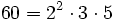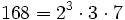# Projective special linear group

(diff) ← Older revision | Latest revision (diff) | Newer revision → (diff)

## Particular cases

### Finite fields

Some facts:

• For$q = 2$,$PSL(n,q) = SL(n,q) = PGL(n,q) = GL(n,q)$. For$q$ a power of two,$PSL(n,q) = SL(n,q)$ but this is not equal to$GL(n,q)$.
• Projective special linear group equals alternating group in only finitely many cases: All those cases are listed in the table below.
• Projective special linear group is simple except for finitely many cases, all of which are listed below.
Size of field Order of matrices Common name for the projective special linear group Order of group Comment$q$ 1 Trivial group$1$ Trivial
2 2 Symmetric group:S3$6 = 2 \cdot 3$ supersolvable but not nilpotent. Not simple.
3 2 Alternating group:A4$12 = 2^2 \cdot 3$ solvable but not supersolvable group. Not simple.
4 2 Alternating group:A5$60 = 2^2 \cdot 3 \cdot 5$ simple non-abelian group of smallest order.
5 2 Alternating group:A5$60 = 2^2 \cdot 3 \cdot 5$ simple non-abelian group of smallest order.
7 2 Projective special linear group:PSL(3,2)$168 = 2^3 \cdot 3 \cdot 7$ simple non-abelian group of second smallest order.
9 2 Alternating group:A6$360 = 2^3 \cdot 3^2 \cdot 5$ simple non-abelian group.
2 3 Projective special linear group:PSL(3,2)$168 = 2^3 \cdot 3 \cdot 7$ simple non-abelian group of second smallest order.
3 3 Projective special linear group:PSL(3,3)$5616 = 2^4 \cdot 3^3 \cdot 13$ simple non-abelian group.